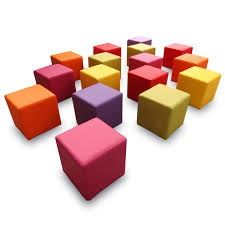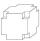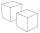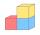# Big cube

Calculate the surface of the cube, which is composed of 64 small cubes with an edge 1 cm long.

S =  96 cm2

### Step-by-step explanation:Did you find an error or inaccuracy? Feel free to write us. Thank you!Tips to related online calculators
Do you want to convert length units?

## Related math problems and questions:

• Cube cornersA small cube with an edge length of 2 cm was cut from each corner of a large cube with an edge length of 10 cm. How many cm3 was the body left from the big cube after cutting the small cubes?
• EightEight small Christmas balls with a radius of 1 cm have the same volume as one large Christmas ball. What has a bigger surface: eight small balls, or one big ball?
• Cube 9What was the cube's original edge length after cutting 39 small cubes with an edge of 2 dm left 200 dm3?
• Two boxes-cubesTwo boxes cube with edges a=38 cm and b = 81 cm is to be replaced by one cube-shaped box (same overall volume). How long will be its edge?
• BallsThree metal balls with volumes V1=71 cm3 V2=78 cm3 and V3=64 cm3 melted into one ball. Determine it's surface area.
• Volume and areaWhat is the volume of a cube which has an area of 361 cm2?
• CubesSurfaces of cubes, one of which has an edge of 48 cm shorter than the other, differ by 36288 dm2. Determine the length of the edges of this cubes.
• Two cubesThe surfaces of two cubes, one of which has an edge of 22 cm longer than the second are differ by 19272 cm2. Calculate the edge length of both cubes.
• Cube cornersThe wooden cube with edge 64 cm was cut in 3 corners of cube with edge 4 cm. How many cubes of edge 4 cm can be even cut?
• Cube 6Volume of the cube is 216 cm3, calculate its surface area.
• Minimum surfaceFind the length, breadth, and height of the cuboid-shaped box with a minimum surface area, into which 50 cuboid-shaped blocks, each with length, breadth, and height equal to 4 cm, 3 cm, and 2 cm respectively, can be packed.
• Prism XThe prism with the edges of the lengths x cm, 2x cm, and 3x cm has volume 20250 cm3. What is the area of the surface of the prism?
• CubesHow many cubes with an edge length of 5 cm may fit in the cube with an inner edge of 0.4 m maximally?
• CalculateCalculate the length of the wall diagonal of the cube with an edge 5 cm long.
• LatheCalculate the percentage of waste if the cube with 53 cm long edge is lathed to cylinder with a maximum volume.
• Three cubesTwo cube-shaped boxes with edges a = 70 cm; b = 90 cm must be replaced by one cube-shaped box. What will be its edge?
• PrismRight-angled prism, whose base is a right triangle with leg a = 3 cm and hypotenuse c = 13 cm, has the same volume as a cube with an edge length of 3 dm. a) Find the height of the prism b) Calculate the surface of the prism c) What percentage of the cube'